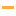# Math Games

Play the best free online Math Games! Check out the Top 100 Math games from PrimaryGames.

#1What Time Is It?Look at the analog clock and tell the time. Then pick the digital clock that shows the same time.

#2Math Lines
#3Fishy Count
#4Tic Tac Toe Comparing NumbersThis game combines the classic game of tic tac toe with math! Choose either the less than, greater than or equal to sign to complete each number comparison problem problem to earn a square on the board. Play a game of tic tac toe against the computer or...

#5Pizza Party FractionsGet ready for a Pizza Party! Click on the fraction that shows the amount of pizza that is left.

#6Math BuzzUse your math skills to protect the queen and collect pollen throughout 5 unique worlds.

#7Animals Dot to Dot Puzzle
#8Math DuckRun and jump in this fun online platform adventure game. Help this cute duck solve math equations to get the key. Use the the key to unlock the door to move on to the next level. You will need to think and move fast while using your addition and...

#9Draw 123Practice tracing each number from 1 to 10. Count objects and learn the word for each number.

#10Time Clock
#11Shape Match Game
#12Math Man
#13Number SnakeHelp the snake slither through the obstacle course. Collect golden circles to add more length to your snake. The snake will get one unit shorter for each number on the blocks. If the you touch a block with a higher number than the size of your snake,...

#14Turkey Tens
#15Crewmate MathSolve each logic puzzle by moving groups of crewmates to the colored boxes. The number of crewmates in each colored box must add up to the total sum given for that box. Practice basic addition skills in this fun online math game.

#16Number Twins
#17Bus Driver's MathYou're the bus driver in this fun math game. Your job is to calculate whether the passengers have paid enough money for the bus fare.

#18Comparing NumbersPractice comparing two numbers between 1 and 10 in this fun online math game. The alligators are hungry! They have a huge appetite and always want to eat the bigger number. Practice saying if one number is less than, greater than, or equal to another...

#19MMMR: Mean, Median, Mode And RangeLearn how to express data and patterns using numbers: mean, median, mode, and range. Use this interactive statistics game to practice calculating and observing data sets.

#20Spring Math MunchmanPractice addition, subtraction, and multiplication in this math game. Gobble up power pellets showing the correct answer to the math problem shown. Don't eat the wrong answer or you will lose a life.